Get instant live expert help with Excel or Google Sheets“My Excelchat expert helped me in less than 20 minutes, saving me what would have been 5 hours of work!”

#### Post your problem and you’ll get expert help in seconds.

Your message must be at least 40 characters
Our professional experts are available now. Your privacy is guaranteed.

# How to Count Text in Excel

Microsoft Word allows you count text, but what about Excel? If you need a count of your text in Excel, you can get this by using the COUNTIF function and then a combination of other functions depending on your desired result.

## How to count text in Excel

If you want to learn how to count text in Excel, you need to use function COUNTIF with the criteria defined using wildcard *, with the formula: `=COUNTIF(range;"*")`. Range is defined cell range where you want to count the text in Excel and wildcard * is criteria for all text occurrences in the defined range.

Some interesting and very useful examples will be covered in this tutorial with the main focus on the COUNTIF function and different usages of this function in text counting. Limitations of COUNTIF function have been covered in this tutorial with an additional explanation of other functions such as SUMPRODUCT/ISNUMBER/FIND functions combination. After this tutorial, you will be able to count text cells in excel, count specific text cells, case sensitive text cells and text cells with multiple criteria defined – which is a very good base for further creative Excel problem-solving.

### Count Text Cells in Excel

Text Cells can be easily found in Excel using COUNTIF or COUNTIFS functions. The COUNTIF function searches text cells based on specific criteria and in the defined range. As in the example below, the defined range is table Name list, and text criteria is defined using wildcard “*”. The formula result is 5, all text cells have been counted. Note that number formatted as text in cell B10 is also counted, but Booleans (TRUE /FALSE) and error (#N/A) are not recognized as text.

The formula for counting text cells:

`=COUNTIF(range;"*")`

For counting non-text cells, the formula should be a little bit changed in criteria part:

`=COUNTIF(range;"<>*")`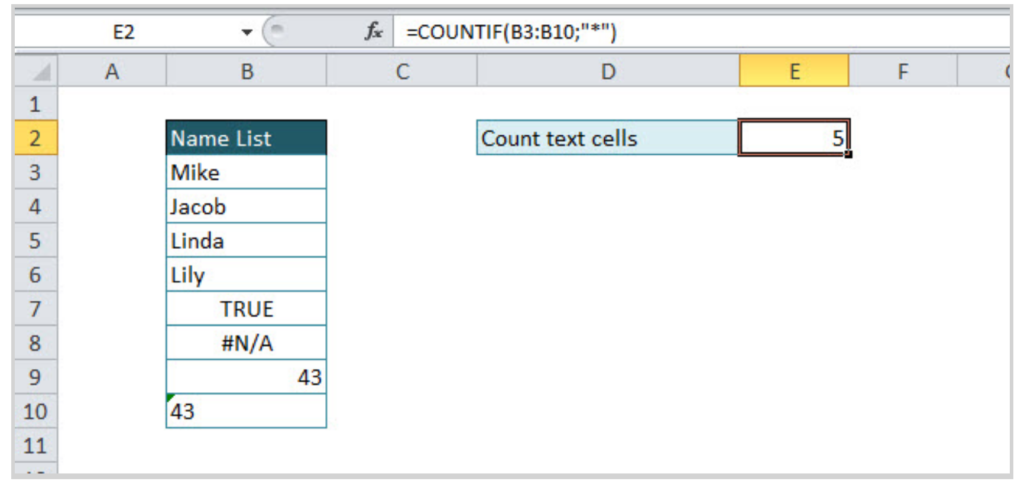If there are several criteria for counting cells, then COUNTIFS function should be used. For example, if we want to count the number of employees from Texas with project number greater than 20, then the function will look like:

`=COUNTIFS(C3:C6;"Texas";D3:D6;">20").`

In criteria range in column State, a specific text criteria is defined under quotations “Texas”. The second criteria is numeric, criteria range is column Number of projects, and criteria is numeric value greater than 20, also under quotations “>20”. If we were looking for exact value, the formula would look like:

`=COUNTIFS(C3:C6;"Texas";D3:D6;20)`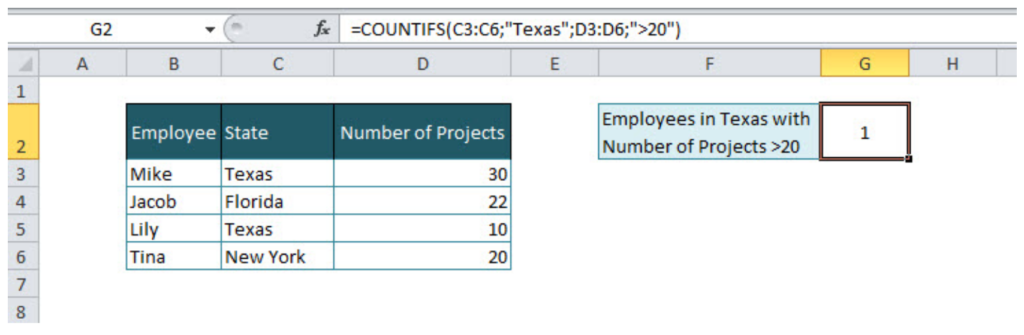### Count Specific Text in Cells

For counting specific text under cells range, COUNTIF function is suitable with the formula:

`=COUNTIF(range;"*text*")`

`=COUNTIF(B3:B9;"*Mike*")`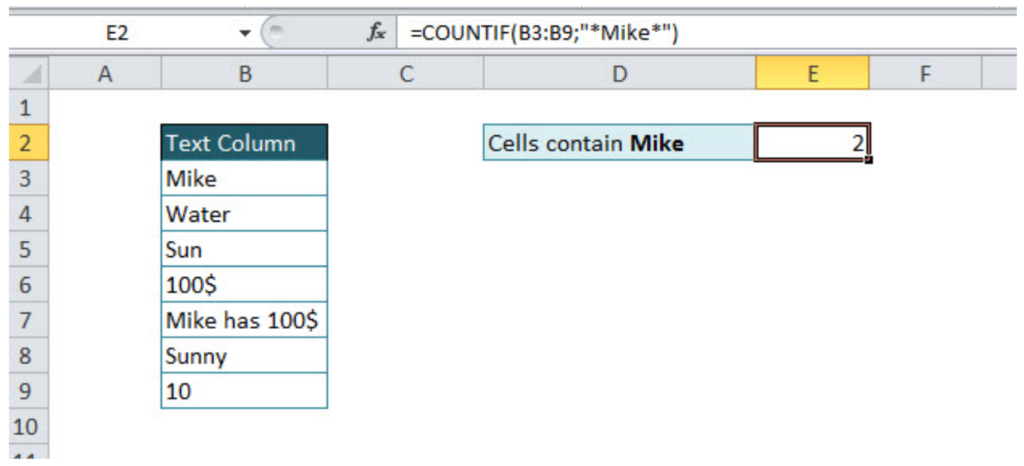The first part of the formula is range and second is text criteria, in our example  “*Mike*”. If wildcard * has not been used before and after criteria text, formula result would have been 1 (Formula would find cells only with word Mike).  Wildcard * before and after criteria text, means that all cells that contain criteria characters will be taken into account. As in another example below with text criteria Sun, three cells were found (sun, Sunny, sun is shining)

`=COUNTIF(B3:B10;"*Sun*")`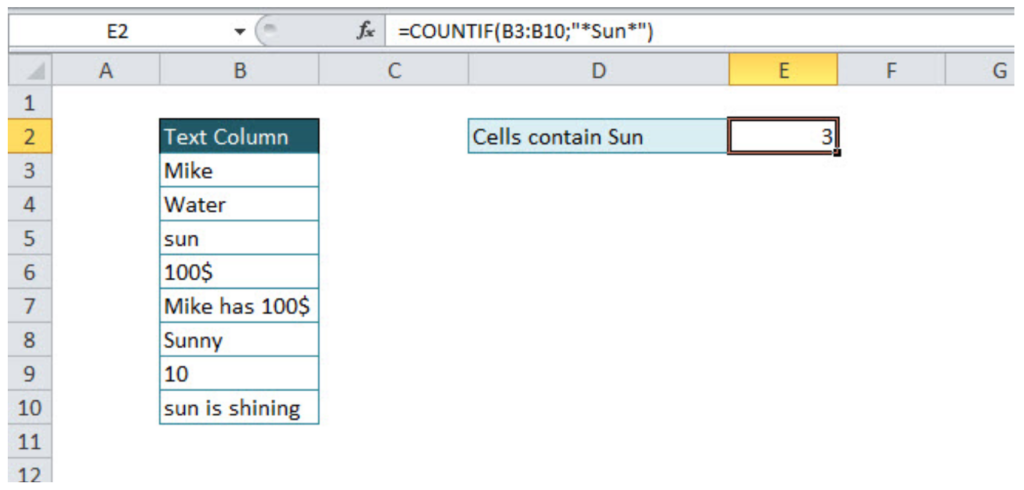Note: The COUNTIF function is not case sensitive, an alternative function for case sensitive text searches is SUMPRODUCT/FIND function combination.

### Count Case Sensitive Specific Text

For a case-sensitive text count, a combination of three formulas should be used: SUMPRODUCT, ISNUMBER and FIND. Let’s look in the example below. If we want to count cells that contain text Sun, case sensitive, COUNTIF function would not be the appropriate solution, instead of this function combination of three functions mentioned above has to be used.

`=SUMPRODUCT(--ISNUMBER(FIND("Sun";B3:B10)))`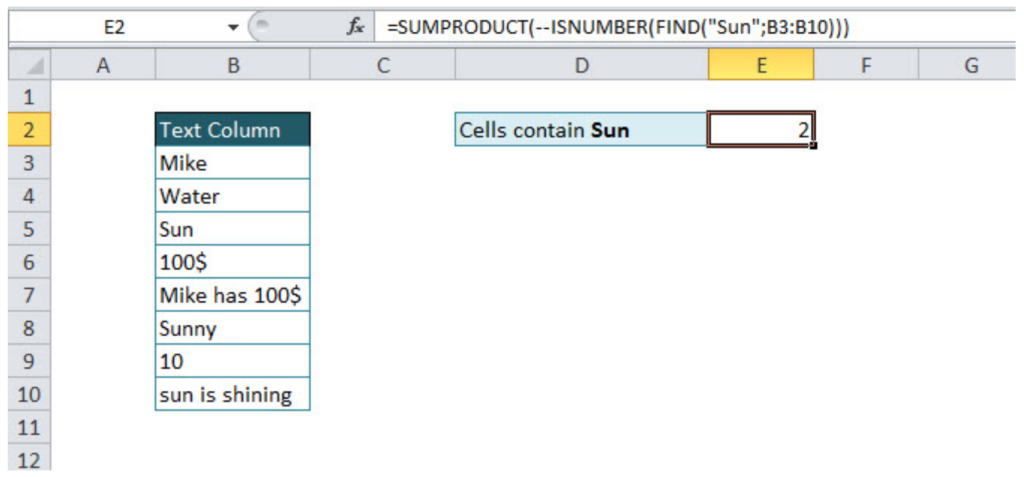We should go through a separate function explanation in order to understand functions combination. FIND function, searches specific text in the defined cell, and returns the number of the starting position of the text used as criteria. This explanation is relevant, if the searching range is just one cell. If we want to use FIND function in a range of the cells, then the combination with SUMPRODUCT function is necessary.

Without ISNUMBER, function combination of FIND and SUMPRODUCT functions would return an error. ISNUMBER function is necessary because whenever FIND function does not match defined criteria, the output will be an error, as in print screen below of the evaluated formula.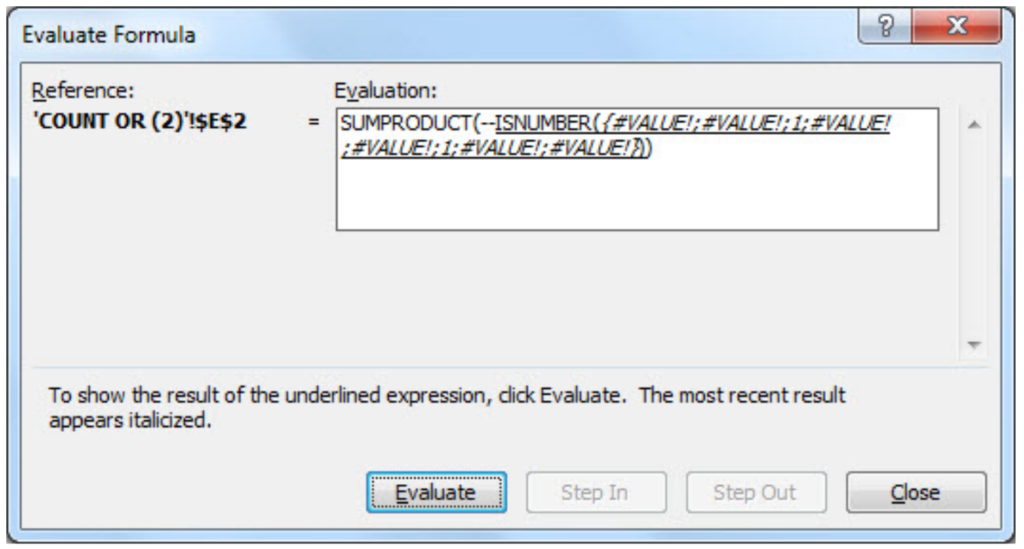In order to change error values with Boolean TRUE/FALSE statement, ISNUMERIC formula should be used (defining numeric values as TRUE, and non-numeric as FALSE, as in print screen below).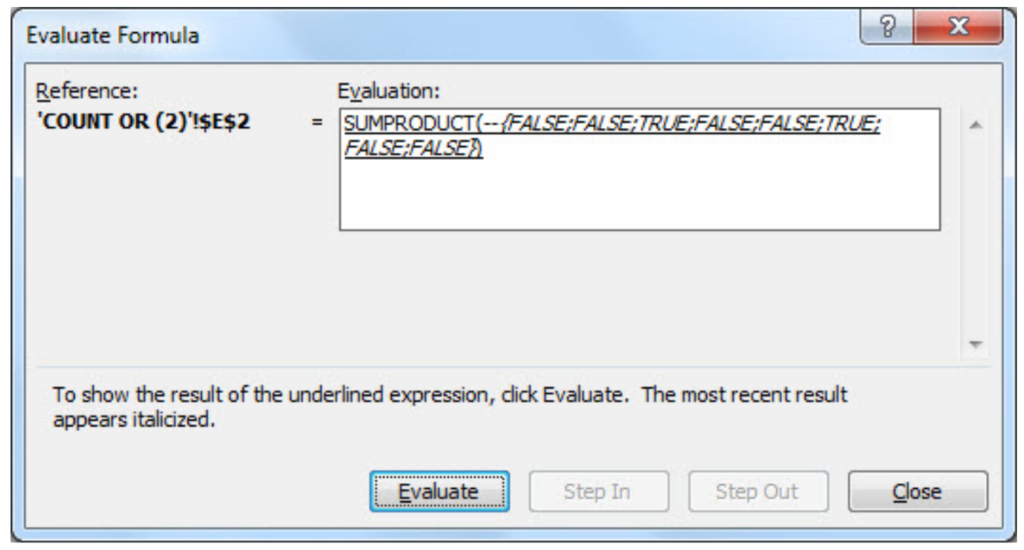You might be wondering what character in SUMPRODUCT function stands for. It converts Boolean values TRUE/FALSE in numeric values 1/0, enabling SUMPRODUCT function to deal with numeric operations (without character — in SUMPRODUCT function, the final result would be 0).

Remember, if you want to count specific text cells that are not case sensitive, COUNTIF function is suitable. For all case sensitive searches combination of SUMPRODUCT/ISNUMBER/FIND functions is appropriate.

### Count Text Cells with Multiple Criteria

If you want to count cells with Multiple criteria, with all criteria acceptable, there is an interesting way of solving that problem, a combination of SUMPRODUCT/ISNUMBER/FIND functions. Please take a look in the example below. We should count all cells that contain either Mike or \$. Tricky part could be the cells that contain both Mike and \$.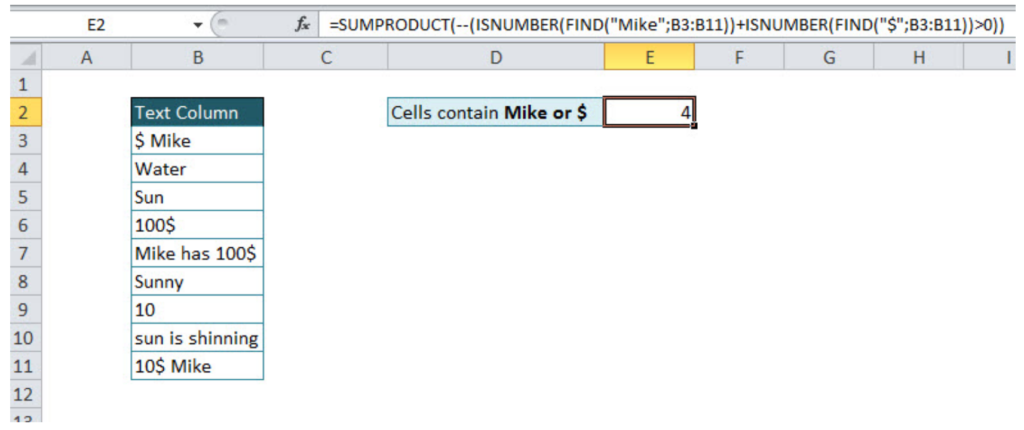`=SUMPRODUCT(--(ISNUMBER(FIND("Mike";B3:B11))+ISNUMBER(FIND("\$";B3:B11))>0))`

Formula just looks complex, in order to be easier for understanding, I will divide it into several steps. Also, knowledge from the previous tutorial point will be necessary for further work, since the combination of FIND, ISNUMBER, and SUMPRODUCT functions have been explained.

In the first part of the function, we loop through the table and find cells that contain Mike:

`=ISNUMBER(FIND("Mike";B3:B11))`

The output of this part of the function will be an array with values  {1;0;0;0;1;0;0;0;1}, number 1, where criteria have been met, and 0, where has not.

In the second part of the function, looping criteria is \$, counting cells containing this value:

`=ISNUMBER(FIND("\$";B3:B11))`

The output of this part of the function will be an array with values  {1;0;0;1;1;0;0;0;1}, number 1, where criteria have been met, and 0, where has not.

Next step is to sum these two arrays, since cell should be counted if any of conditions is fulfilled:

`=ISNUMBER(FIND("Mike";B3:B11))+ISNUMBER(FIND("\$";B3:B11))`

The output of this step is {2;0;0;1;2;0;0;0;2}, the number  greater than 0 means that one of the condition has been met (2 – both conditions, 1 – one condition)

Without function part >0, the final function would double count cells that met both conditions and the final result would be 7 (sum of all array numbers). In order to avoid it, in the formula should be added >0:

`=ISNUMBER(FIND("Mike";B3:B11))+ISNUMBER(FIND("\$";B3:B11))>0`

The output of this step is array {1;0;0;1;1;0;0;0;1}, the previous array has been checked and only values greater than 0 are TRUE (in an array have value 1), and others are FALSE (in an array have value 0).

Final output of the formula is the sum of the final array values, 4.

Looks very confusing, but after several usages, you will become familiar with this functions.

At the end, we will cover one more multiple criteria text count function, already mentioned in the tutorial, COUNTIFS function. In order to distinguish the usage of functions mentioned above and COUNTIFS function, two words are enough OR/AND. If you want to count text cells with multiple criteria but all conditions have to be met at the same time, then COUNTIFS function is appropriate. If at least one condition should be met, then the combination of function explained above is suitable.

Look at the example below, the number of cells that contain both Mike and \$ is easily calculated with COUNTIFS function:

`=COUNTIFS(range1;"*text1*";range2;"*text2*")`

`=COUNTIFS(B3:B11;"*Mike*";B3:B11;"*\$*")`

In the defined range, function counts only cells where both conditions have been met. The final result is 3.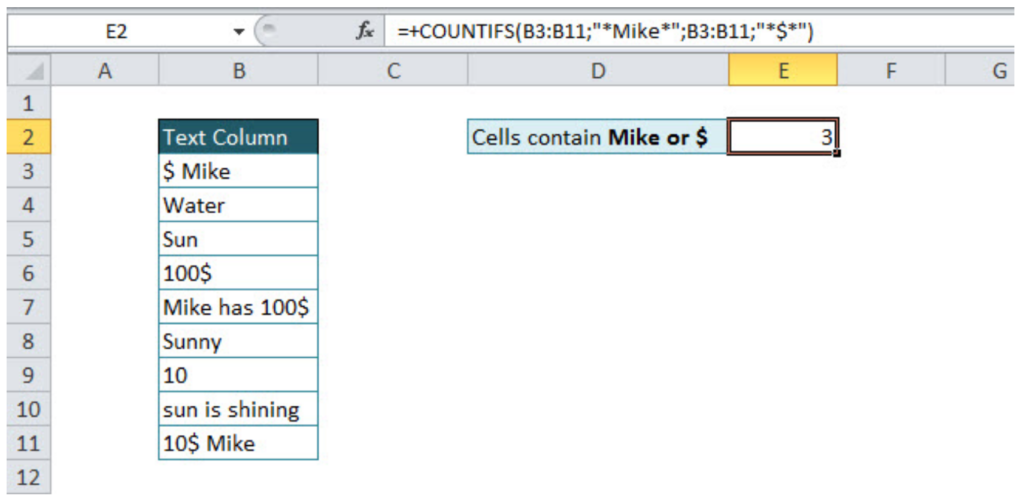Still need some help with Excel formatting or have other questions about Excel? Connect with a live Excel expert here for some 1 on 1 help. Your first session is always free.

### Did this post not answer your question? Get a solution from connecting with the expert.Another blog reader asked this question today on Excelchat:
Related blogs
Solution examplesI want to display a range of cells if they meet a specific criteria. I want to display a name IF it does not equal one of three options AND there are any numbers contained in a range of cells
Solved by G. F. in 60 minscan you teach me the steps to create a nested if function, and to nest an AND function inside of an IF function?
Solved by A. Q. in 22 minsI have a list or people who are ranked in numbers from 3 to 6 I need to recognize the contents of each multiple cells and create an equivalent letter. for that value into another column. e.g. Cell E1 = 3 to show in new cell that row (H1) the letter "C" I have multiple rows with different values in column "E" Can this be done?
Solved by I. J. in 30 minsCan you re edit this formula for me =IFERROR(IF(LEN(MID("RHS",1,FIND("RHS",B4,1)))=1,LEFT(B4,3),MID("RHS",1,FIND("RHS",B4,1))),MID("LHS",1,FIND("LHS",B4,1)))
Solved by S. L. in 40 minsIF(AVERAGE(C6+E6+G6)>=5000,TRUE,FALSE) I am using AND function and it is not giving me the correct answer
Solved by G. J. in 24 mins## Subscribe to Excelchat.coAnother blog reader asked this question today on Excelchat: